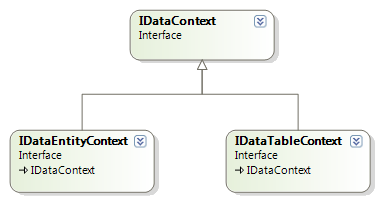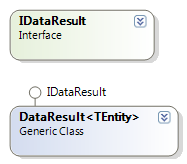# [转载][原创].NET 业务框架开发实战之六 DAL的重构

.NET 业务框架开发实战之六 DAL的重构

1. 确定DAL的接口的定义。

Richard思考了之前对DAL的设计，在此他做了一些改进。IDataEntityContext接口的和系列文章中定义的一些方法差不多，但是做了修改。其中有一点要提的就是：ICriteria就是所有条 件对象要实现的接口(查询对象也是条件对象的一种)。例如，可以根据相应的条件删除，更新数据。代码

/// <summary>
/// 所有的 数据实体执行者实现这个借口
/// </summary>
public interface IDataEntityContext:IDataContext
{
<TEntity>(TEntity entity) where TEntity : IDataEntity;
List
<TEntity> Add<TEntity>(List<TEntity> entityList) where TEntity : IDataEntity;

bool Update<TEntity>(TEntity entity) where TEntity : IDataEntity;
bool Update<TEntity>(List<TEntity> entityList) where TEntity : IDataEntity;
bool Update(ICriteria condiftion, object value);

bool Delete<TEntity>(TEntity entity) where TEntity : IDataEntity;
bool Delete<TEntity>(List<TEntity> entityList) where TEntity : IDataEntity;
bool Delete(ICriteria condition);

int GetCount(ICriteria condition);
List
<TEntity> Query<TEntity>(ICriteria condition);
List
<TEntity> Query<TEntity>(ICriteria condition, int pageIndex, int pageSize, ref int entityCount) where TEntity : IDataEntity;
List
<object> Query(ICriteria condiftion);
}代码

/// <summary>
/// 所有的 条件对象都要从这个接口继承
/// </summary>
public interface ICriteria
{
string Name { get; set; }
bool IsCache { get; set; }
bool IsTransaction { get; set; }
}代码

/// <summary>
/// 数据提 供者要实现的借口
/// </summary>
public interface IDataProvider
{
DataResult
<TEntity> Add<TEntity>(TEntity entity) where TEntity : IDataEntity;
DataResult
<TEntity> Add<TEntity>(List<TEntity> entityList) where TEntity : IDataEntity;

DataResult<TEntity> Update<TEntity>(TEntity entity) where TEntity : IDataEntity;
DataResult
<TEntity> Update<TEntity>(List<TEntity> entityList) where TEntity : IDataEntity;
bool Update(ICriteria condiftion, object value);

DataResult<TEntity> Delete<TEntity>(TEntity entity) where TEntity : IDataEntity;
DataResult
<TEntity> Delete<TEntity>(List<TEntity> entityList) where TEntity : IDataEntity;
bool Delete(ICriteria condiftion);

int GetCount(ICriteria condition);

DataResult<TEntity> GetOne<TEntity>(ICriteria condition) where TEntity : IDataEntity;
DataResult
<TEntity> GetList<TEntity>(ICriteria condition) where TEntity : IDataEntity;
DataResult
<TEntity> GetPageData<TEntity>(ICriteria condition, int pageIndex, int pageSize, ref int entityCount) where TEntity : IDataEntity;
List
<object> GetCustomData(ICriteria condiftion);
}DAL的设计就到这里，下一篇文章就开始讲述对业务层的一些思考。

http://www.cnblogs.com/yanyangtian

### 觉得文章有用就打赏一下文章作者

#### 支付宝扫一扫打赏#### 微信扫一扫打赏• QQ咨询
• 回顶